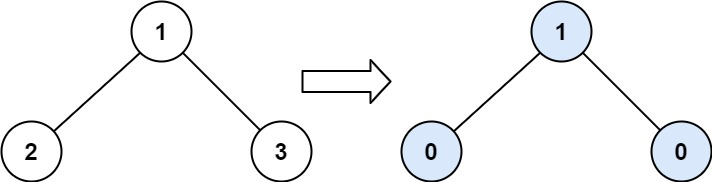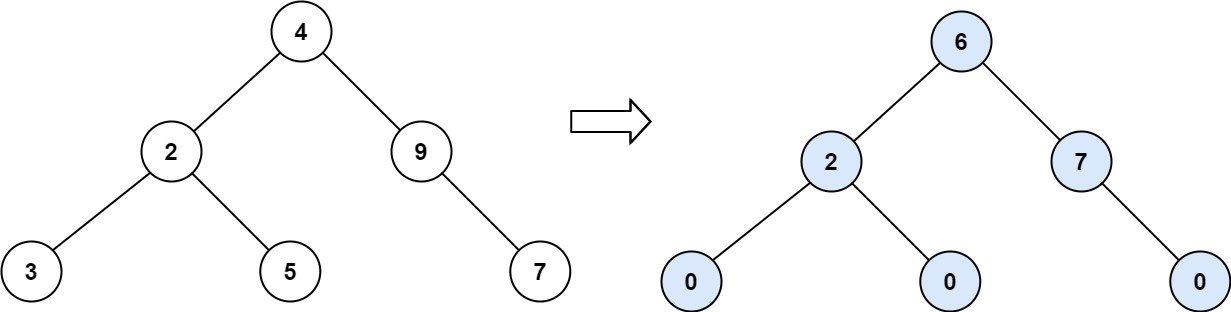# leetcode 563题

Posted by franki on November 14, 2022

## leetcode 563 反转字符串中的单词 III``````输入：root = [1,2,3]

````````````输入：root = [4,2,9,3,5,null,7]

``````

### 代码

``````/**
* @param {TreeNode} root
* @return {number}
*/
function findTilt(root) {
let sum = 0;

const dfs = (node) => {
if (node === null) {
return 0;
}

const left = dfs(node.left);
const right = dfs(node.right);
sum += Math.abs(left - right);
return node.val + left + right;
};

dfs(root);
return sum;
}
``````

• 时间复杂度: O(logN)
• 空间复杂度：O(1)